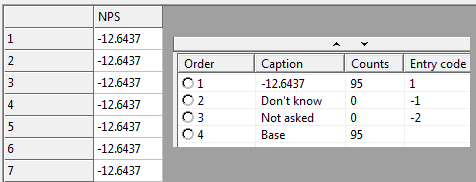Scroll

# Accessing Counts (Calculating NPS in a Variable)

Follow
 Summary This article is one in a series of articles that will go through examples of using aggregate script syntax new to Askia 5.3.5.0. Specifically, this article demonstrates how to return the counts from one or more codes in your questions and then use them to carry out simple calculated scores that are stored in a variable. Applies to Askia Analyse. Written for Researchers, Data Processors, Analysts. Keywords Aggregated script, Calculated variables, Numeric by script, Find all values of a script, Net Promoter Score, NPS, Temporary variables, Data object, Value, Counts, Groups, Nets.

The .qes file and portfolio containing the example discussed is attached: Aggregated Scripts Examples 3.rar.

Let’s look at how we can return counts from a question:

A simple example is to return the exact same counts as another question in a calculated variable.

In the example .qes file, create a variable (find all values of a script) then use:  q4.Value.

You’ll see that the code index is returned as the captions along with the relevant counts: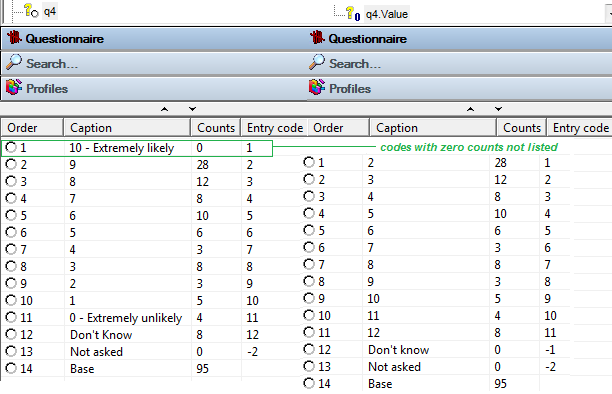We can return the counts of individual codes in a question by using the following syntax

q4.Data.Counts()[N]

Where N is the index of the code for which you want to return the counts e.g.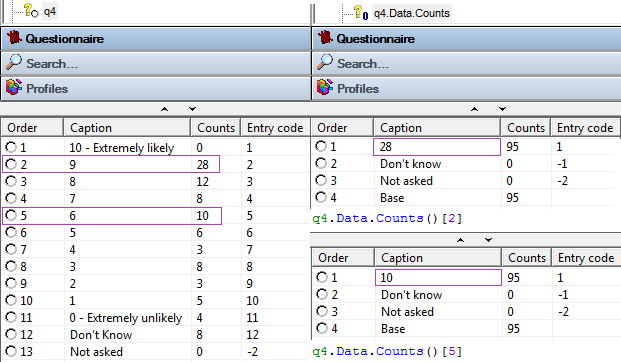You can also return grouped counts. The syntax is slightly different:

q4.Data.Counts({Set})[True]

The codes you want to group are now defined as a set in the parentheses following .Counts. In the square bracket you simply place 1 or True.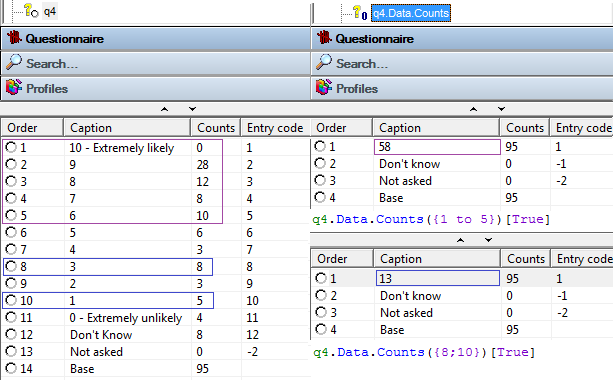Now I will show how we can store our Net promoter Score (NPS) in a calculated variable.

You might normally set it up like this, with NPS tied to a code-frame, and worked out using a calculated response: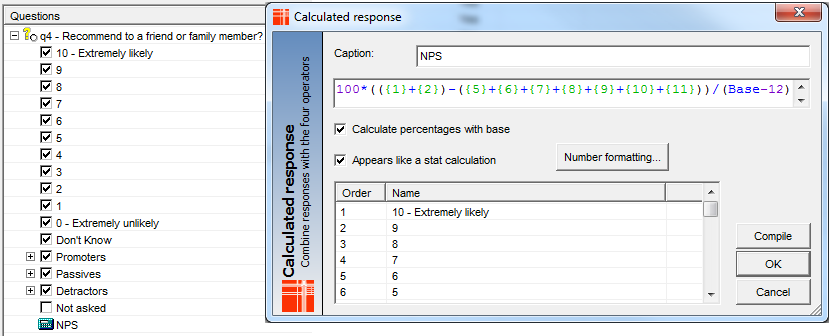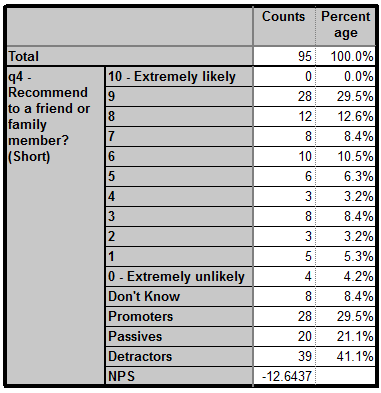It might be useful to store NPS in a numeric variable to use for other calculations. Create a variable like below: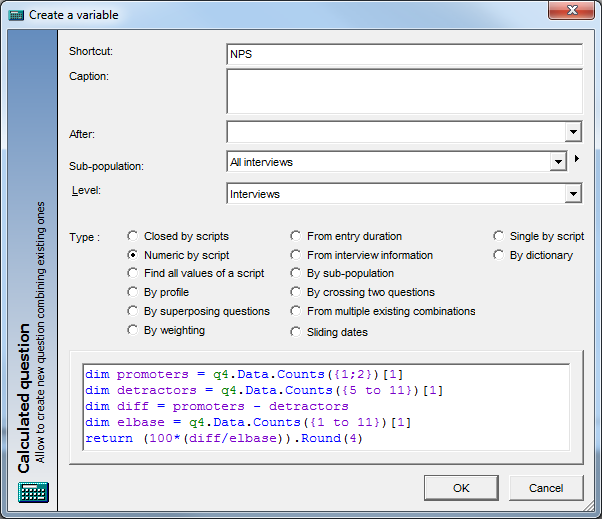q4.Data.Counts({1;2})

This creates the number of counts of those answering 10 or 9 at q4 (promoters)

q4.Data.Counts({5 to 11})

This creates the number of counts of those answering 0 to 6 at q4 (detractors)

We subtract the two and store it in our diff temporary variable

q4.Data.Counts({1 to 11})

This works out the eligible base for the NPS calculation i.e. only those giving a score (elbase)

We then return (100*(diff/elbase)).Round(4) which gives us our NPS.

You can also store it in a 'Find all values of a script' calculation. Either will now store the (aggregated) Net Promoter Score for every respondent.